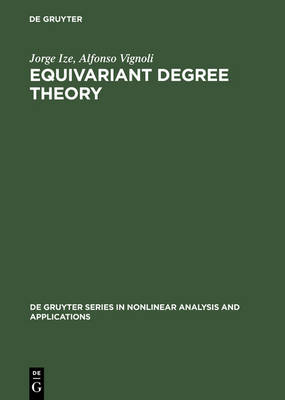•Equivariant Degree Theory - De Gruyter Series in Nonlinear Analysis & Applications (Hardback)

(author), (author)
£136.50
Hardback 380 Pages / Published: 16/04/2003
• We can order this

Usually dispatched within 3 weeks

This book presents a new degree theory for maps which commute with a group of symmetries. This degree is no longer a single integer but an element of the group of equivariant homotopy classes of maps between two spheres and depends on the orbit types of the spaces. The authors develop completely the theory and applications of this degree in a self-contained presentation starting with only elementary facts. The first chapter explains the basic tools of representation theory, homotopy theory and differential equations needed in the text. Then the degree is defined and its main abstract properties are derived. The next part is devoted to the study of equivariant homotopy groups of spheres and to the classification of equivariant maps in the case of abelian actions. These groups are explicitely computed and the effects of symmetry breaking, products and composition are thorougly studied. The last part deals with computations of the equivariant index of an isolated orbit and of an isolated loop of stationary points. Here differential equations in a variety of situations are considered: symmetry breaking, forcing, period doubling, twisted orbits, first integrals, gradients etc. Periodic solutions of Hamiltonian systems, in particular spring-pendulum systems, are studied as well as Hopf bifurcation for all these situations.

Publisher: De Gruyter
ISBN: 9783110175509
Number of pages: 380
Weight: 754 g
Dimensions: 240 x 170 x 25 mm
Edition: Reprint 2012